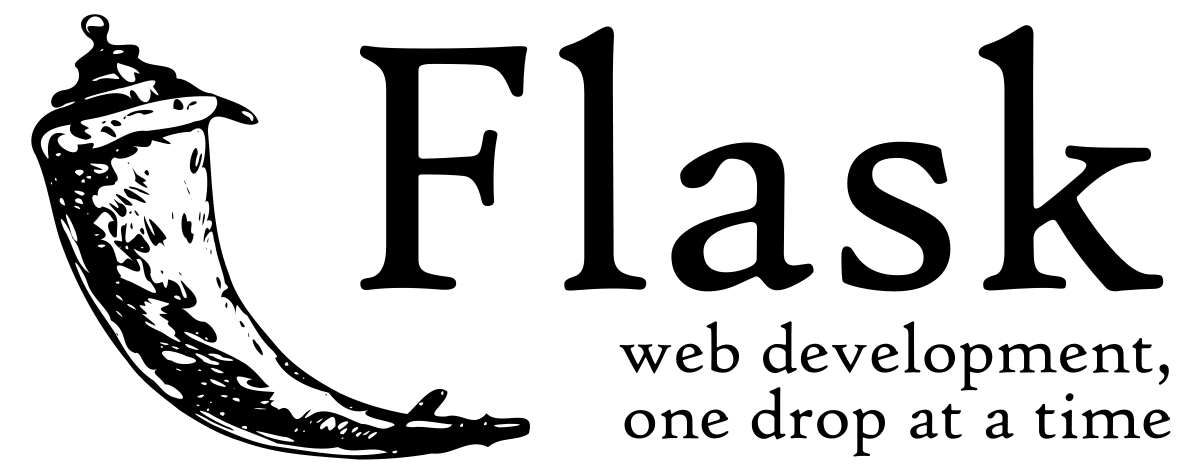# Create an API with Flask

Use Flask to create APIs in Python and deploy them on Saturn CloudTry this example in seconds on Saturn Cloud

## Overview

Flask is a microframework for creating APIs in Python. An API is a way for programs to communicate with each other. They work similarly to websites, but instead of a human typing in a url and getting an HTML page back, a program can send a similar request to a URL and get different types of data back.

This API example runs a linear regression on historical house prices and outputs the predicted house price based on this model when you hit a specific endpoint.

## Creating the API

All the API code is contained in a file called “app.py.” There is also a basic HTML front end for the API in the “templates” folder.

To deploy this dashboard on Saturn Cloud, call `flask run --host=0.0.0.0 --port=8000` as the command in a Saturn Cloud deployment. For users to access the server, the host value must be `"0.0.0.0"` and the port number `"8000"`. `8000` is the only port exposed on Saturn Cloud deployments.

See Saturn Cloud docs for more detailed instructions on deploying this and other dashboards.

### Import the Libraries

This exercise uses scikit-learn and Flask to create a simple API.

``````from flask import Flask, render_template, request
import pandas as pd
from sklearn.linear_model import LinearRegression
``````

### Load the Data and Create the Model

First, we load the housing price data from S3 into a pandas DataFrame. This data is then passed into a scikit-learn model that uses the number of bedrooms and the year the house was built to predict the sale price of the home. This model is relatively simple, so we create it when we start the API server, but you could also load a more complex pre-trained model.

``````df = pd.read_csv(
"https://saturn-public-data.s3.us-east-2.amazonaws.com/examples/dashboard/housePriceData.csv"
)
linear_regression = LinearRegression().fit(df[["BedroomAbvGr", "YearBuilt"]], df["SalePrice"])
``````

### Define the Endpoints

After creating the model, define the endpoints of the API. Here we are defining two endpoints: `/predict` and `/`. The `/` endpoint function runs when you hit the base url of the API. This endpoint renders the HTML defined in the “templates” folder.

The `/predict` endpoint runs the model prediction function. It takes two arguments: the number of bedrooms of the house and the year the house was built. You run this endpoint by filling out the HTML form and clicking Submit. This function predicts sales price based on the model and returns a string with the prediction.

``````@app.route("/")
def index():
return render_template("index.html")

@app.route("/predict", methods=["POST"])
def predict():
bedrooms = int(request.form["bedrooms"])
year_built = int(request.form["year_built"])

if not ((1 <= bedrooms <= 8) and (1872 <= year_built <= 2100)):
return render_template(
"index.html",
data="Invalid entry. Please enter a number of bedrooms between 1 and 8 and a year built between 1872 and 2100.",
)

input = pd.DataFrame([[bedrooms, year_built]], columns=["BedroomAbvGr", "YearBuilt"])
prediction = linear_regression.predict(input)

return render_template("index.html", data=f"The house price is: \${int(prediction)}.")
``````

### Define the Form

The form is a basic HTML form that calls the API when the Submit button is pressed. Flask requires this to be stored in the “templates” folder.

``````<!DOCTYPE html>
<html>

<title>House Price Prediction</title>

<body>
<h1>House Price Prediction</h1>
<form action="{{url_for('predict')}}" method="POST">
Bedrooms: <input type="number" name='bedrooms' value=1><br>
Year of Build: <input type="number" name='year_built' value=1872><br><br>
<input type="submit" value="predict" class="submit">
</form>
<br>
<p> {{data}}</p>
</body>

</html>
``````

### Run the API

You then need to only load the app code to git and link the code appropriately to a Saturn Cloud deployment.

For more examples of creating APIs and dashboards with Python check out the other Saturn Cloud examples.